Joy of Problem Solving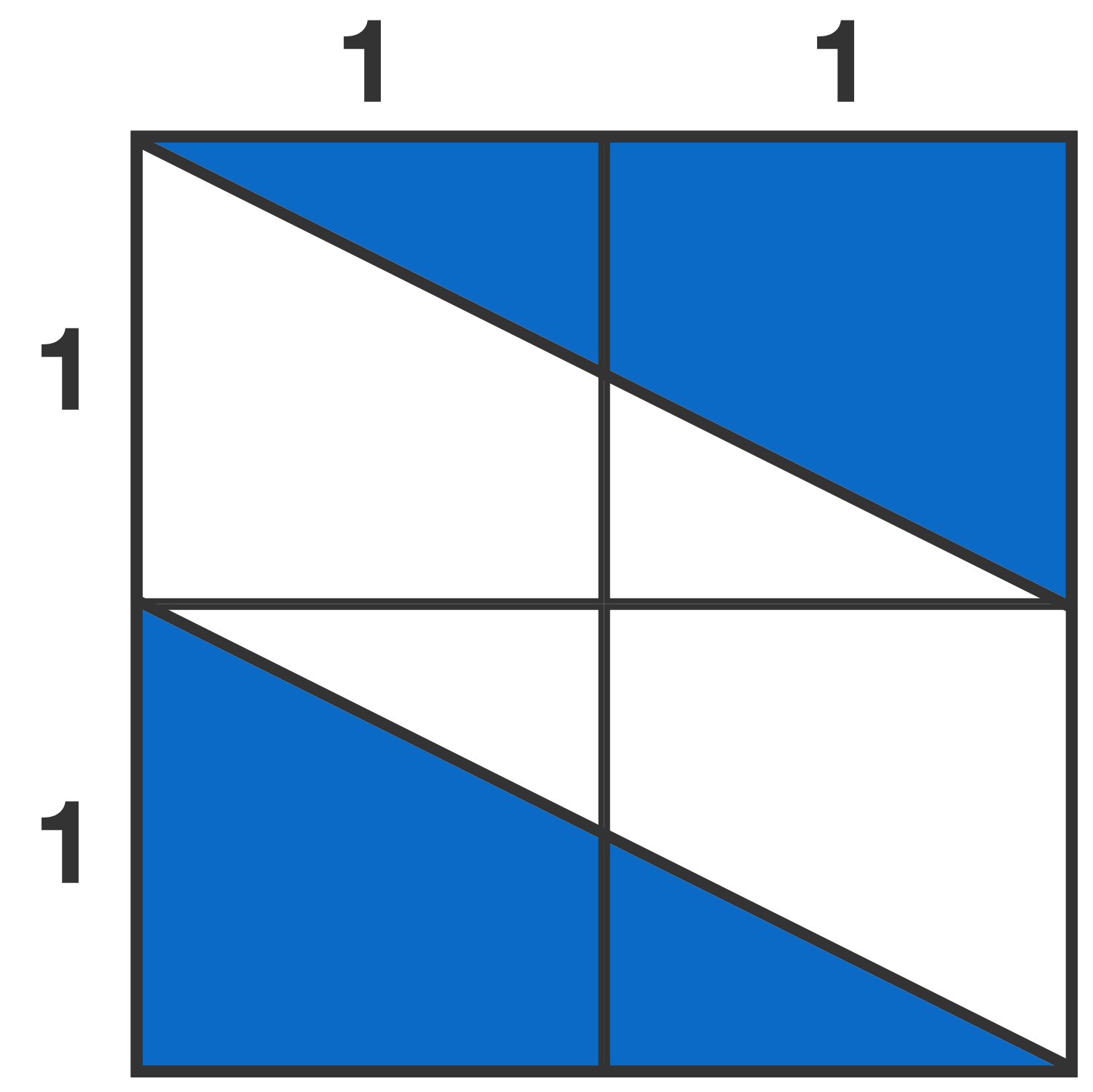The figure consists of 4 unit squares. What is the area of the portion shaded blue?

Geometric Shortcuts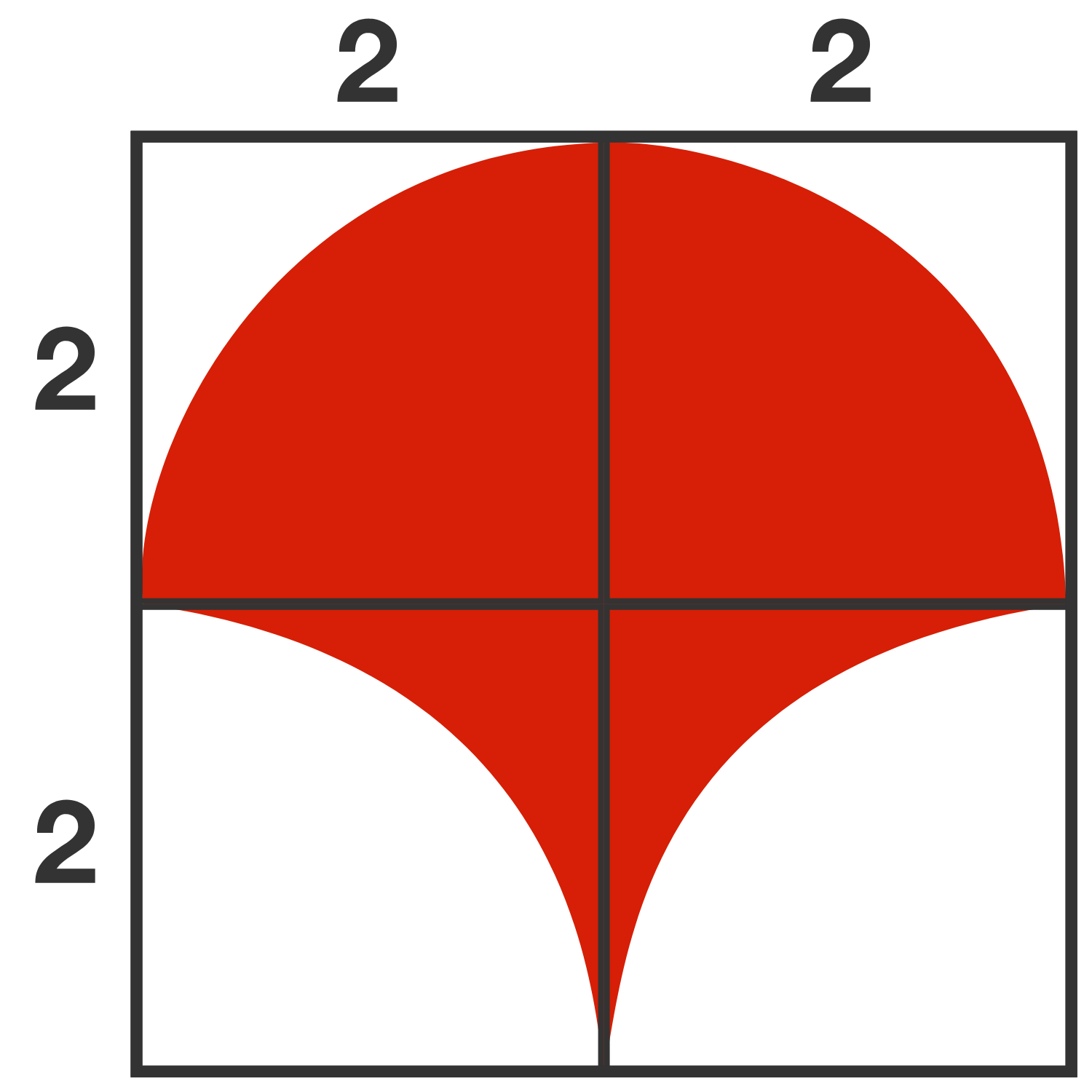What is the area of the red figure? (All arcs shown are of circles with a radius of 2.)

Geometric Shortcuts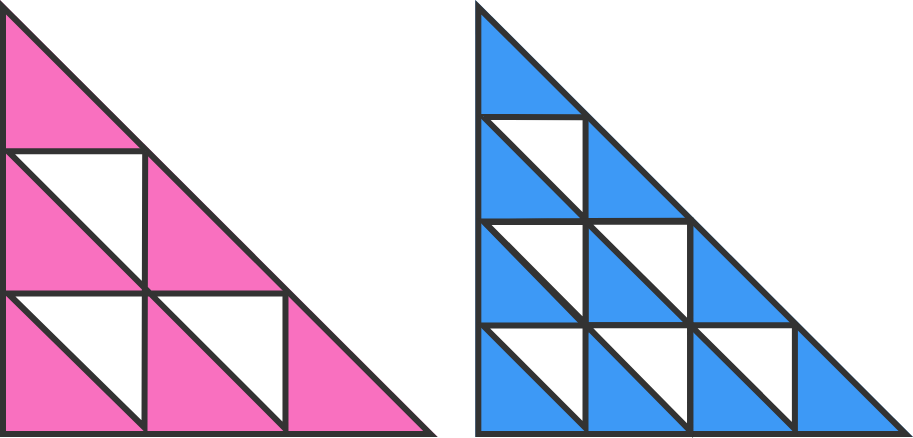The large right triangles are identical. Which is larger, the pink area on the left or the blue area on the right?

All internal triangles are similar to the larger triangles.

Geometric Shortcuts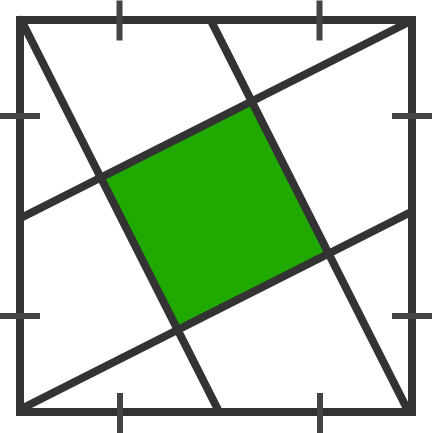A square has line segments connecting corners to midpoints, as shown. What fraction of the larger square is colored green?

Geometric Shortcuts

In the square below, two semicircles are overlapping in a symmetrical pattern. Which is greater: the area shaded blue or the area shaded white?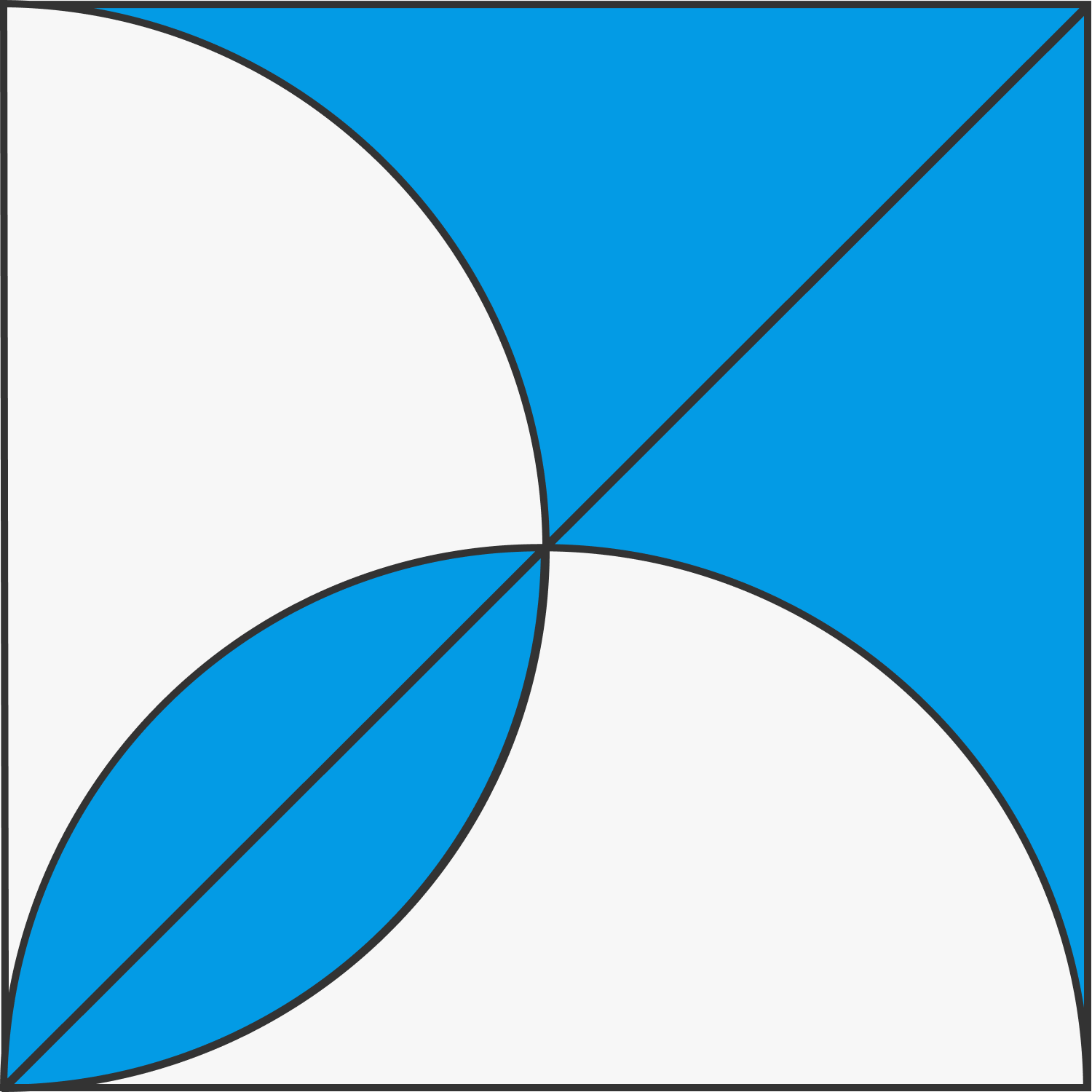×